# 9. Hybrid Orbitals.ppt

23. Feb 2023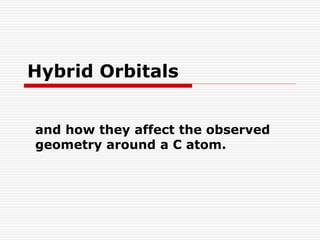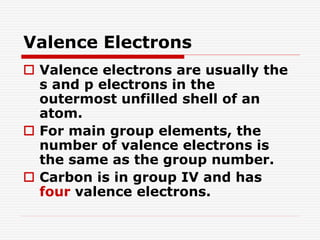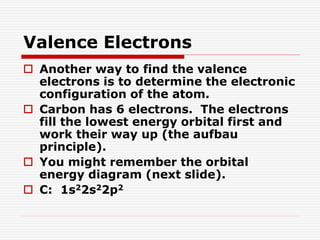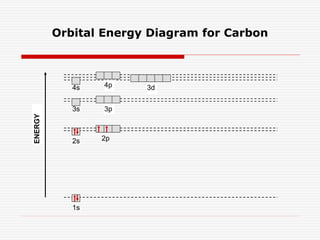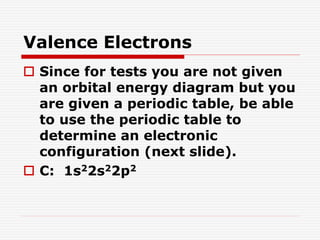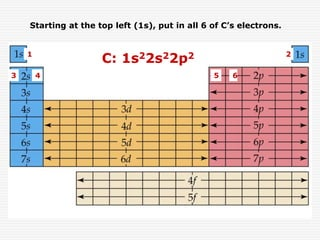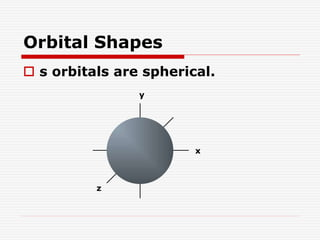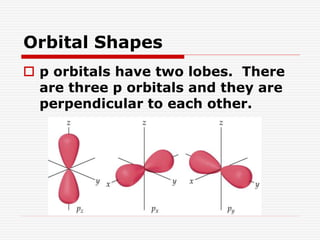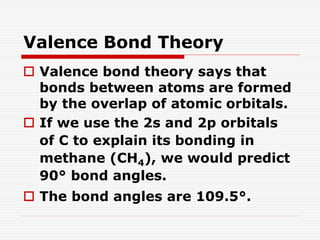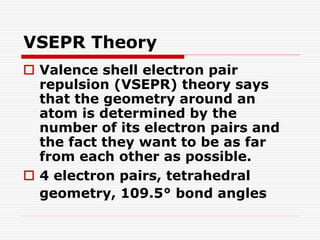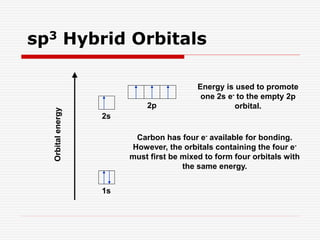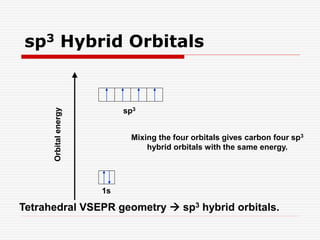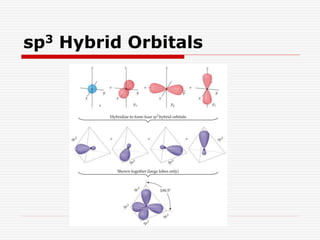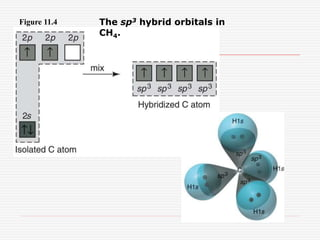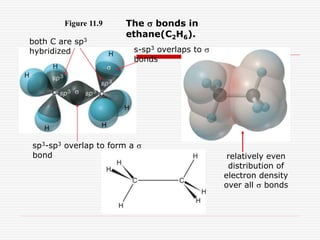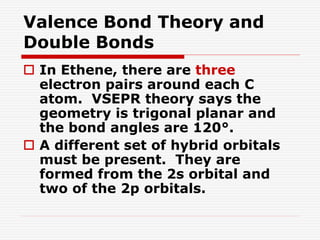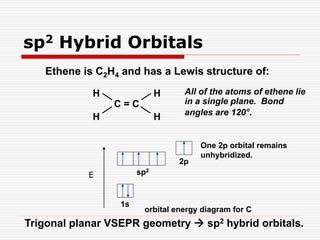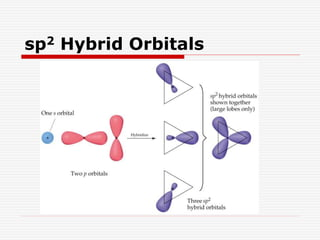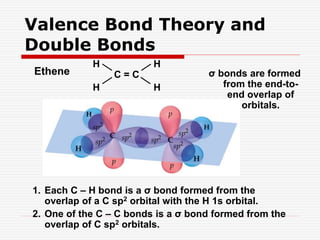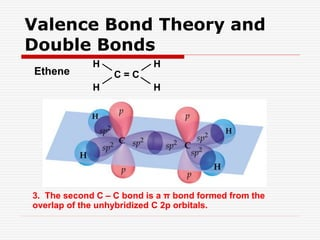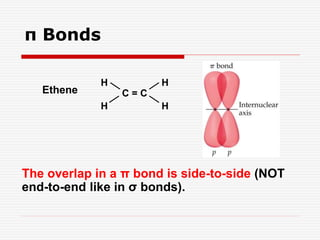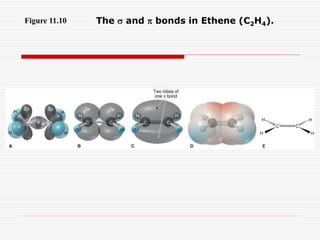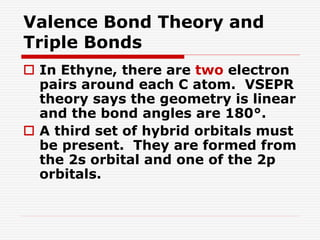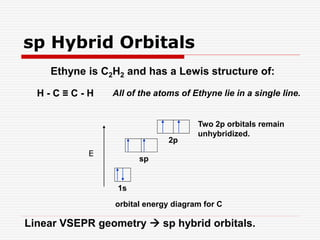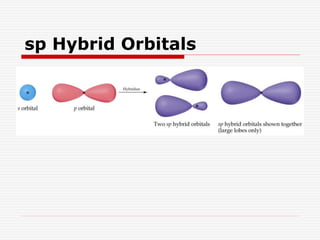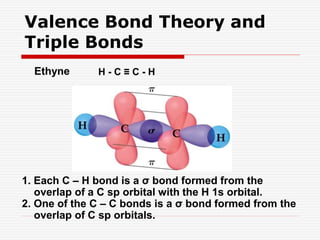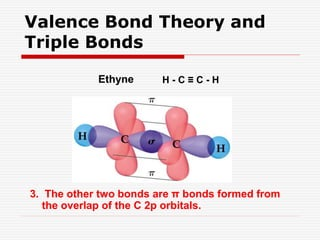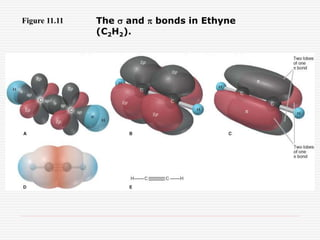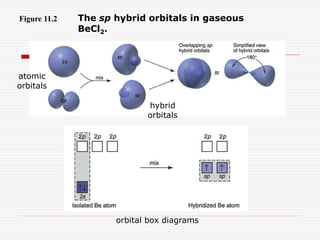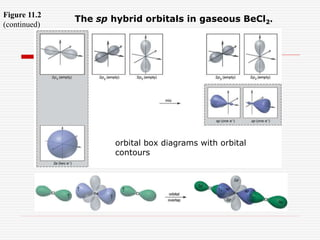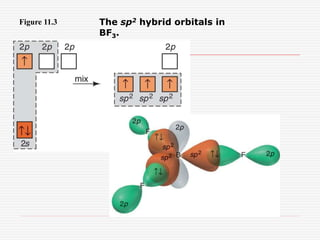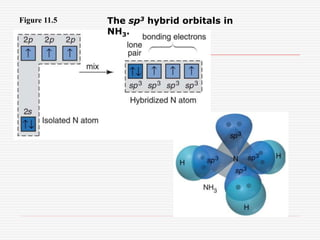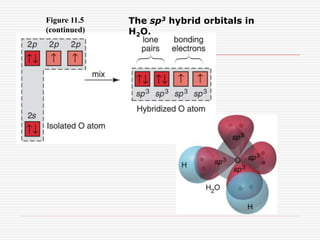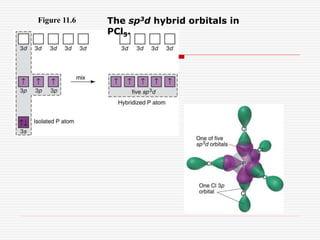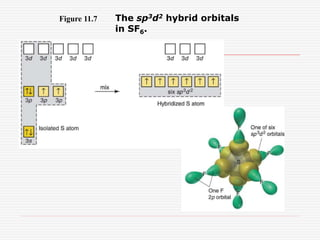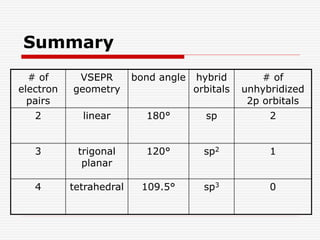1 von 36

### 9. Hybrid Orbitals.ppt

• 1. Hybrid Orbitals and how they affect the observed geometry around a C atom.
• 2. Valence Electrons  Valence electrons are usually the s and p electrons in the outermost unfilled shell of an atom.  For main group elements, the number of valence electrons is the same as the group number.  Carbon is in group IV and has four valence electrons.
• 3. Valence Electrons  Another way to find the valence electrons is to determine the electronic configuration of the atom.  Carbon has 6 electrons. The electrons fill the lowest energy orbital first and work their way up (the aufbau principle).  You might remember the orbital energy diagram (next slide).  C: 1s22s22p2
• 4. Orbital Energy Diagram for Carbon 1s 3s 4s 2s 2p 4p 3p 3d ENERGY
• 5. Valence Electrons  Since for tests you are not given an orbital energy diagram but you are given a periodic table, be able to use the periodic table to determine an electronic configuration (next slide).  C: 1s22s22p2
• 6. C: 1s22s22p2 Starting at the top left (1s), put in all 6 of C’s electrons. 1 2 3 4 5 6
• 7. Orbital Shapes  s orbitals are spherical. y x z
• 8. Orbital Shapes  p orbitals have two lobes. There are three p orbitals and they are perpendicular to each other.
• 9. Valence Bond Theory  Valence bond theory says that bonds between atoms are formed by the overlap of atomic orbitals.  If we use the 2s and 2p orbitals of C to explain its bonding in methane (CH4), we would predict 90° bond angles.  The bond angles are 109.5°.
• 10. VSEPR Theory  Valence shell electron pair repulsion (VSEPR) theory says that the geometry around an atom is determined by the number of its electron pairs and the fact they want to be as far from each other as possible.  4 electron pairs, tetrahedral geometry, 109.5° bond angles
• 11. sp3 Hybrid Orbitals 1s 2s 2p Orbital energy Energy is used to promote one 2s e- to the empty 2p orbital. Carbon has four e- available for bonding. However, the orbitals containing the four e- must first be mixed to form four orbitals with the same energy.
• 12. sp3 Hybrid Orbitals 1s sp3 Orbital energy Mixing the four orbitals gives carbon four sp3 hybrid orbitals with the same energy. Tetrahedral VSEPR geometry  sp3 hybrid orbitals.
• 13. sp3 Hybrid Orbitals
• 14. Figure 11.4 The sp3 hybrid orbitals in CH4.
• 15. Figure 11.9 The s bonds in ethane(C2H6). both C are sp3 hybridized s-sp3 overlaps to s bonds sp3-sp3 overlap to form a s bond relatively even distribution of electron density over all s bonds
• 16. Valence Bond Theory and Double Bonds  In Ethene, there are three electron pairs around each C atom. VSEPR theory says the geometry is trigonal planar and the bond angles are 120°.  A different set of hybrid orbitals must be present. They are formed from the 2s orbital and two of the 2p orbitals.
• 17. Ethene is C2H4 and has a Lewis structure of: 1s sp2 2p orbital energy diagram for C One 2p orbital remains unhybridized. H H H H C = C All of the atoms of ethene lie in a single plane. Bond angles are 120°. sp2 Hybrid Orbitals Trigonal planar VSEPR geometry  sp2 hybrid orbitals. E
• 18. sp2 Hybrid Orbitals
• 19. Ethene H H H H C = C Valence Bond Theory and Double Bonds 1. Each C – H bond is a σ bond formed from the overlap of a C sp2 orbital with the H 1s orbital. 2. One of the C – C bonds is a σ bond formed from the overlap of C sp2 orbitals. σ bonds are formed from the end-to- end overlap of orbitals.
• 20. Ethene H H H H C = C 3. The second C – C bond is a π bond formed from the overlap of the unhybridized C 2p orbitals. Valence Bond Theory and Double Bonds
• 21. Ethene H H H H C = C The overlap in a π bond is side-to-side (NOT end-to-end like in σ bonds). π Bonds
• 22. Figure 11.10 The s and p bonds in Ethene (C2H4).
• 23. Valence Bond Theory and Triple Bonds  In Ethyne, there are two electron pairs around each C atom. VSEPR theory says the geometry is linear and the bond angles are 180°.  A third set of hybrid orbitals must be present. They are formed from the 2s orbital and one of the 2p orbitals.
• 24. Ethyne is C2H2 and has a Lewis structure of: 1s sp 2p orbital energy diagram for C Two 2p orbitals remain unhybridized. H - C ≡ C - H All of the atoms of Ethyne lie in a single line. E Linear VSEPR geometry  sp hybrid orbitals. sp Hybrid Orbitals
• 25. sp Hybrid Orbitals
• 26. Valence Bond Theory and Triple Bonds Ethyne H - C ≡ C - H 1. Each C – H bond is a σ bond formed from the overlap of a C sp orbital with the H 1s orbital. 2. One of the C – C bonds is a σ bond formed from the overlap of C sp orbitals.
• 27. Ethyne H - C ≡ C - H 3. The other two bonds are π bonds formed from the overlap of the C 2p orbitals. Valence Bond Theory and Triple Bonds
• 28. Figure 11.11 The s and p bonds in Ethyne (C2H2).
• 29. Figure 11.2 The sp hybrid orbitals in gaseous BeCl2. atomic orbitals hybrid orbitals orbital box diagrams
• 30. Figure 11.2 (continued) The sp hybrid orbitals in gaseous BeCl2. orbital box diagrams with orbital contours
• 31. Figure 11.3 The sp2 hybrid orbitals in BF3.
• 32. Figure 11.5 The sp3 hybrid orbitals in NH3.
• 33. Figure 11.5 (continued) The sp3 hybrid orbitals in H2O.
• 34. Figure 11.6 The sp3d hybrid orbitals in PCl5.
• 35. Figure 11.7 The sp3d2 hybrid orbitals in SF6.
• 36. Summary # of electron pairs VSEPR geometry bond angle hybrid orbitals # of unhybridized 2p orbitals 2 linear 180° sp 2 3 trigonal planar 120° sp2 1 4 tetrahedral 109.5° sp3 0